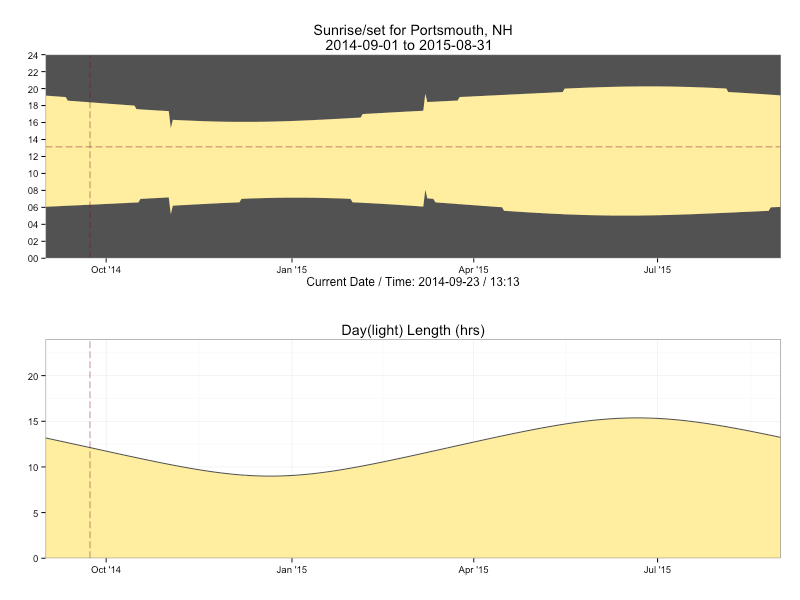# Seeing the (day)light with R

The arrival of the autumnal equinox foreshadows the reality of longer nights and shorter days here in the northeast US. We can both see that reality and distract ourselves from it at the same time by firing up RStudio (or your favorite editor) and taking a look at the sunrise & sunset times based on our map coordinates using some functions from the R {maptools} package.

The `sunriset` function takes in a lat/lon pair, a range of dates and whether we want sunrise or sunset calculated and returns when those ephemeral events occur. For example, we can see the sunrise time for Portsmouth, NH on Christmas day this year (2014) via:

``````library(maptools)

# these functions need the lat/lon in an unusual format
portsmouth <- matrix(c(-70.762553, 43.071755), nrow=1)
for_date <- as.POSIXct("2014-12-25", tz="America/New_York")
sunriset(portsmouth, for_date, direction="sunrise", POSIXct.out=TRUE)

##         day_frac                time
## newlon 0.3007444 2014-12-25 07:13:04
``````

We can pass in a vector of dates, to this function, and that means we’ll have data points we can work with to visualize this change. Let’s wrap the sequence generation into a function of our own and extract:

• sunrise
• sunset
• solar noon
• # hours of daylight

for every day in the sequence, returning the result as a data frame.

``````# adapted from http://r.789695.n4.nabble.com/maptools-sunrise-sunset-function-td874148.html
ephemeris <- function(lat, lon, date, span=1, tz="UTC") {

# convert to the format we need
lon.lat <- matrix(c(lon, lat), nrow=1)

# make our sequence - using noon gets us around daylight saving time issues
day <- as.POSIXct(date, tz=tz)
sequence <- seq(from=day, length.out=span , by="days")

# get our data
sunrise <- sunriset(lon.lat, sequence, direction="sunrise", POSIXct.out=TRUE)
sunset <- sunriset(lon.lat, sequence, direction="sunset", POSIXct.out=TRUE)
solar_noon <- solarnoon(lon.lat, sequence, POSIXct.out=TRUE)

# build a data frame from the vectors
data.frame(date=as.Date(sunrise\$time),
sunrise=as.numeric(format(sunrise\$time, "%H%M")),
solarnoon=as.numeric(format(solar_noon\$time, "%H%M")),
sunset=as.numeric(format(sunset\$time, "%H%M")),
day_length=as.numeric(sunset\$time-sunrise\$time))

}
``````

Now we can take a look at these values over 10 days near All Hallows Eve:

``````ephemeris(43.071755, -70.762553, "2014-10-31", 10, tz="America/New_York")

##          date sunrise solarnoon sunset day_length
## 1  2014-10-31     716      1226   1736  10.332477
## 2  2014-11-01     717      1226   1734  10.289145
## 3  2014-11-02     518      1026   1533  10.246169
## 4  2014-11-03     620      1126   1632  10.203563
## 5  2014-11-04     621      1126   1631  10.161346
## 6  2014-11-05     622      1126   1629  10.119535
## 7  2014-11-06     624      1126   1628  10.078148
## 8  2014-11-07     625      1126   1627  10.037204
## 9  2014-11-08     626      1126   1626   9.996721
## 10 2014-11-09     627      1126   1625   9.956719
``````

We now have everything we need to visualize the seasonal daylight changes. We’ll use `ggplot` (with some help from the `grid` package) and build a two panel graph, one that gives us a “ribbon” view of what hours of the day are in daylight and the other just showing the changes in the total number of hours of daylight available during the day. We’ll build the function so that it will:

• optionally show the current date/time (`TRUE` by default)
• optionally show when solar noon is (`FALSE` by default)
• optionally plot the graphs (`TRUE` by default)
• return an `arrangeGrob` of the charts in the event we want to use them in other charts
``````library(ggplot2)
library(scales)
library(gridExtra)

# create two formatter functions for the x-axis display

# for graph #1 y-axis
time_format <- function(hrmn) substr(sprintf("%04d", hrmn),1,2)

# for graph #2 y-axis

daylight <- function(lat, lon, place, start_date, span=2, tz="UTC",
show_solar_noon=FALSE, show_now=TRUE, plot=TRUE) {

stopifnot(span>=2) # really doesn't make much sense to plot 1 value

srss <- ephemeris(lat, lon, start_date, span, tz)

x_label = ""

gg <- gg + geom_ribbon(aes(ymin=sunrise, ymax=sunset), fill="#ffeda0")

if (show_solar_noon) gg <- gg + geom_line(aes(y=solarnoon), color="#fd8d3c")

if (show_now) {
gg <- gg + geom_vline(xintercept=as.numeric(as.Date(Sys.time())), color="#800026", linetype="longdash", size=0.25)
gg <- gg + geom_hline(yintercept=as.numeric(format(Sys.time(), "%H%M")), color="#800026", linetype="longdash", size=0.25)
x_label = sprintf("Current Date / Time: %s", format(Sys.time(), "%Y-%m-%d / %H:%M"))
}

gg <- gg + scale_x_date(expand=c(0,0), labels=date_format("%b '%y"))
gg <- gg + scale_y_continuous(labels=time_format, limits=c(0,2400), breaks=seq(0, 2400, 200), expand=c(0,0))
gg <- gg + labs(x=x_label, y="",
title=sprintf("Sunrise/set for %s\n%s ", place, paste0(range(srss\$date), sep=" ", collapse="to ")))
gg <- gg + theme_bw()
gg <- gg + theme(panel.background=element_rect(fill="#525252"))
gg <- gg + theme(panel.grid=element_blank())

gg1 <- gg1 + geom_area(fill="#ffeda0")
gg1 <- gg1 + geom_line(color="#525252")

if (show_now) gg1 <- gg1 + geom_vline(xintercept=as.numeric(as.Date(Sys.time())), color="#800026", linetype="longdash", size=0.25)

gg1 <- gg1 + scale_x_date(expand=c(0,0), labels=date_format("%b '%y"))
gg1 <- gg1 + scale_y_continuous(labels=pad5, limits=c(0,24), expand=c(0,0))
gg1 <- gg1 + labs(x="", y="", title="Day(light) Length (hrs)")
gg1 <- gg1 + theme_bw()

if (plot) grid.arrange(gg, gg1, nrow=2)

arrangeGrob(gg, gg1, nrow=2)

}
``````

We can test our our new function using the same location and graph the sunlight data for a year starting September 1, 2014 (select graph for full-size version):

``````daylight(43.071755, -70.762553, "Portsmouth, NH", "2014-09-01", 365, tz="America/New_York")
``````With the longer nights approaching we can further enhance the plotting function to add markers for solstices and perhaps even make a new version that compares sunlight across different geographical locations.

Complete code example is in this gist.Amazon Author Page

## 4 Comments →Seeing the (day)light with R

1.Gene

Funny, I did something similar a while back. http://geneorama.com/sunshine-in-chicago/
I hate daylight savings! What’s wrong with having the natural day / night cycle?

2.czep

Excellent work. I discovered the maptools package and found this to be the best tutorial on the subject. Thank you!

This site uses Akismet to reduce spam. Learn how your comment data is processed.